Next: Iteration
Prev: Warm Up Questions

# One-Dimensional Dynamical Systems

## Part 2: Logistic Growth Model

The squirrel model in the introduction is called the exponential growth model. Suppose the current number of squirrels is p0, and pn denotes the number of squirrels after n years. Then we can construct the orbit p0, p1, p2, p3, ... by iterating

f(x) =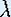x.

We can even find an explicit formula for pn, namely:

pn =n p0.

The name exponential growth model comes from the fact that n is in the exponent.

### Nonlinear dynamics

We have derived a model for population growth,

pn+1 - pn = (- 1) pn (1 -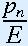).

that depends on a 'saturation' number E and the growth rate parameter. If we substitute pn = E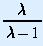xn, and write xn+1 = f(x) and xn = x, then the logistic growth model reduces to the simple dynamical system

f(x) =x (1 - x).

Notice that the parameter E has disappeared. In fact, the variable x =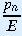*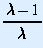does not stand for the total number of squirrels in a specific year. We can think of x as representing a 'normalized' population. Since x is equal totimes a constant, it can be thought of as expressing the population of squirrels as a fraction of some adjusted saturation value.

Next: IterationThe Geometry Center Home Page